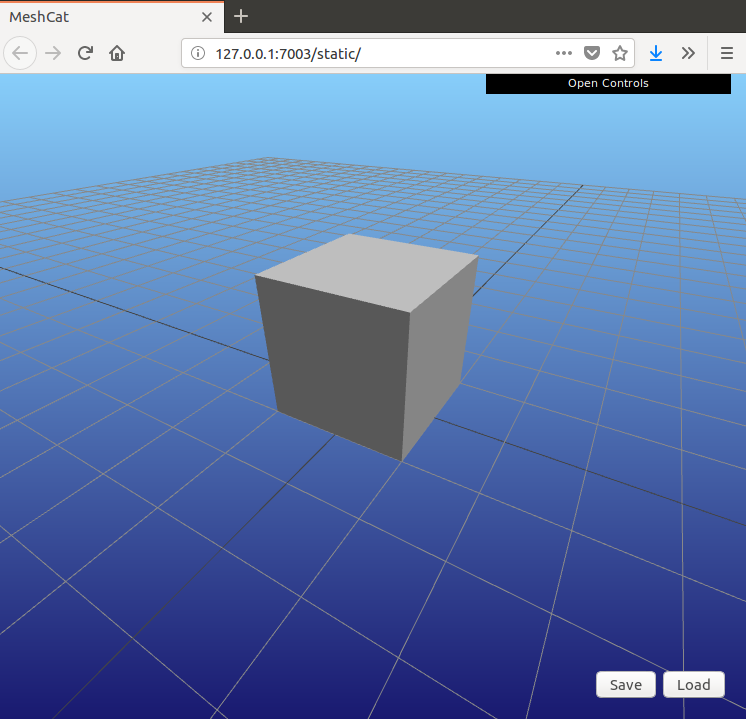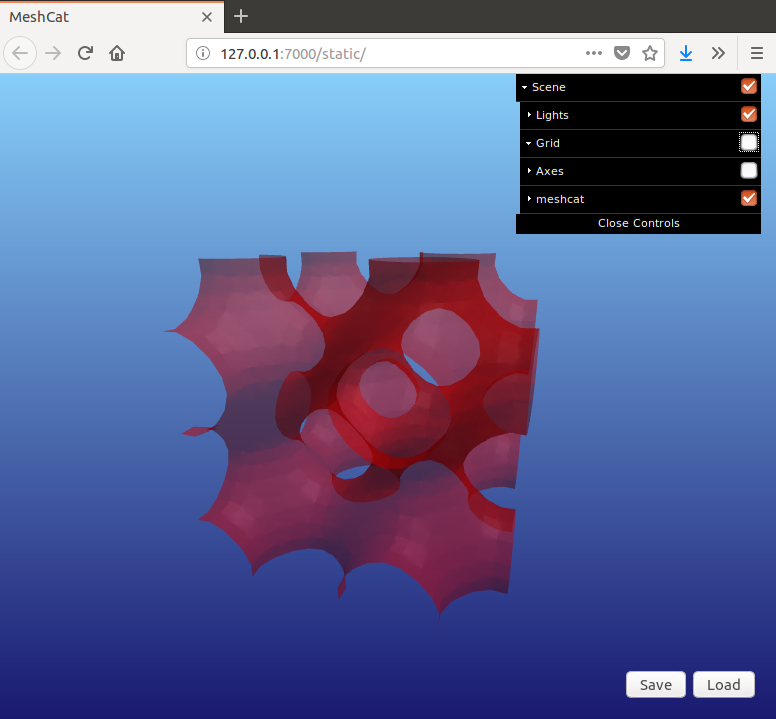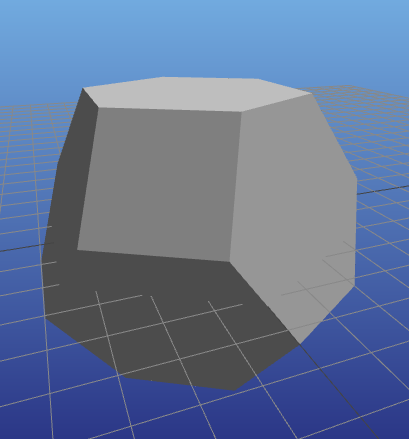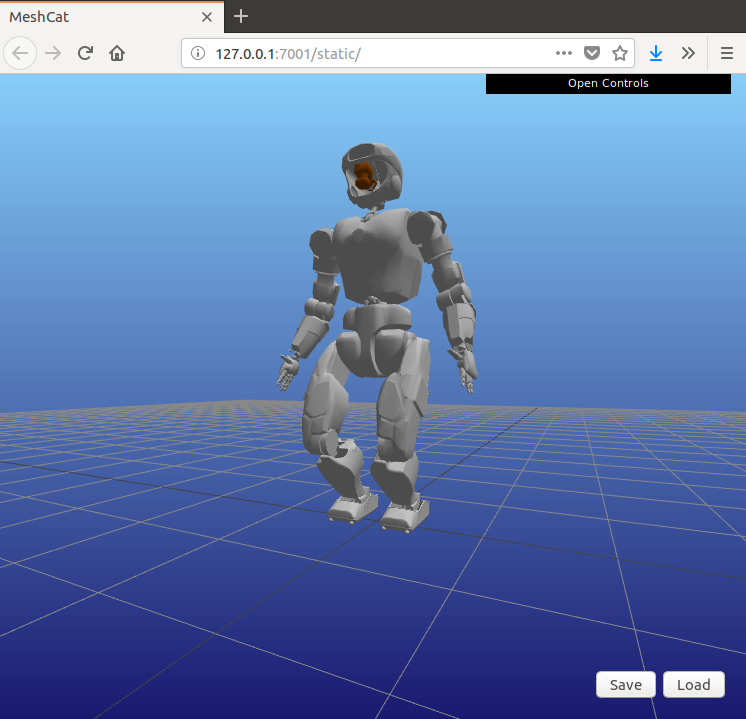## MeshCat.jl

WebGL-based 3D visualizer in Julia
Popularity
207 Stars
Updated Last
4 Months Ago
Started In
February 2018

# MeshCat.jl: Julia bindings to the MeshCat WebGL viewer

MeshCat is a remotely-controllable 3D viewer, built on top of three.js. The viewer contains a tree of objects and transformations (i.e. a scene graph) and allows those objects and transformations to be added and manipulated with simple commands. This makes it easy to create 3D visualizations of geometries, mechanisms, and robots. MeshCat.jl runs on macOS, Linux, and Windows.

The MeshCat viewer runs entirely in the browser, with no external dependencies. All files are served locally, so no internet connection is required. Communication between the browser and your Julia code is managed by HTTP.jl. That means that MeshCat should work:

As much as possible, MeshCat.jl tries to use existing implementations of its fundamental types. In particular, we use:

That means that MeshCat should play well with other tools in the JuliaGeometry ecosystem like MeshIO.jl, Meshing.jl, etc.

# Demos

## Basic Usage

For detailed examples of usage, check out demo.ipynb.

## Animation

To learn about the animation system (introduced in MeshCat.jl v0.2.0), see animation.ipynb.

# Related Projects

MeshCat.jl is a successor to DrakeVisualizer.jl, and the interface is quite similar (with the exception that we use `setobject!` instead of `setgeometry!`). The primary difference is that DrakeVisualizer required Director, LCM, and VTK, all of which could be difficult to install, while MeshCat just needs a web browser. MeshCat also has better support for materials, textures, point clouds, and complex meshes.

You may also want to check out:

# Examples

### Create a visualizer and open it

```using MeshCat
vis = Visualizer()
open(vis)

## In an IJulia/Jupyter notebook, you can also do:
# IJuliaCell(vis)```

### Cube

```using GeometryBasics
using CoordinateTransformations

setobject!(vis, HyperRectangle(Vec(0., 0, 0), Vec(1., 1, 1)))
settransform!(vis, Translation(-0.5, -0.5, 0))```### Point Clouds

```using ColorTypes
verts = rand(Point3f, 100_000)
colors = [RGB(p...) for p in verts]
setobject!(vis, PointCloud(verts, colors))```### Contours

Warning Following the transition from `GeometryTypes` to `GeometryBasics`, this example is no longer working because the `SignedDistanceField` function is not included in `GeometryBasics`. See #239.

```# Visualize a mesh from the level set of a function
using Meshing
f = x -> sum(sin, 5 * x)
sdf = SignedDistanceField(f, HyperRectangle(Vec(-1, -1, -1), Vec(2, 2, 2)))
mesh = HomogenousMesh(sdf, MarchingTetrahedra())
setobject!(vis, mesh,
MeshPhongMaterial(color=RGBA{Float32}(1, 0, 0, 0.5)))```### Polyhedra

See here for a notebook with the example.

```# Visualize the permutahedron of order 4 using Polyhedra.jl
using Combinatorics, Polyhedra
v = vrep(collect(permutations([0, 1, 2, 3])))
using CDDLib
p4 = polyhedron(v, CDDLib.Library())

# Project that polyhedron down to 3 dimensions for visualization
v1 = [1, -1,  0,  0]
v2 = [1,  1, -2,  0]
v3 = [1,  1,  1, -3]
p3 = project(p4, [v1 v2 v3])

# Show the result
setobject!(vis, Polyhedra.Mesh(p3))```### Mechanisms### Required Packages

View all packages